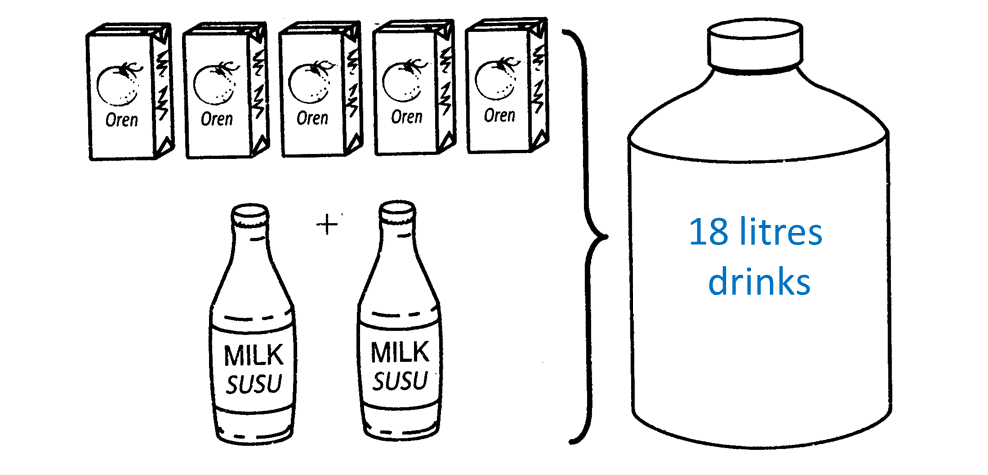# 6.4.5 Linear Equations, PT3 Focus Practice

 
   
   
Question 14:
   
It is given that + 2y = 4 and x + 6y = –4.
   
Calculate the value of x.
   

 
   
Solution:
   
Using Elimination method
   
+ 2y = 4 ——– (1)
   
+ 6y = –4 ——- (2)
   
(1) × 3:  3x + 6y = 12 ——– (3)
   
x + 6y = –4 ——- (2)
   
(3) – (2): 2= 12 – (–4)
   
2x = 16
   
x = 8
 
 
 
 

Question 15:
Calculate the value of x and of y that satisfy the following simultaneous linear equations:
2x + y = 8
x + 4y = 5

Solution:

 
    
Question 16:
 
Diagram below shows 5 boxes of orange juice and 2 bottles of milk. Both orange juice and milk are mixed to produce 18 litres drink.(a) Based on the above situation, write a linear equation.
 (b) If 9 litres of drinks is produced by using 2 boxes of orange juice and 2 bottles of milk, find the volume, in litre, of orange juice in each box.
 
 Solution:
 
Let j be the number of boxes of orange juice and m be the number of bottles of milk.
 (a)
 5j + 2m = 18
 
 (b)
           2j+2m=9  …………. (    1   )           5j+2m=18 …………. (    2   )           (    2   )(    1   ):           3j=9           j=    9    3      =3                      3 litres of orange juice in each box.